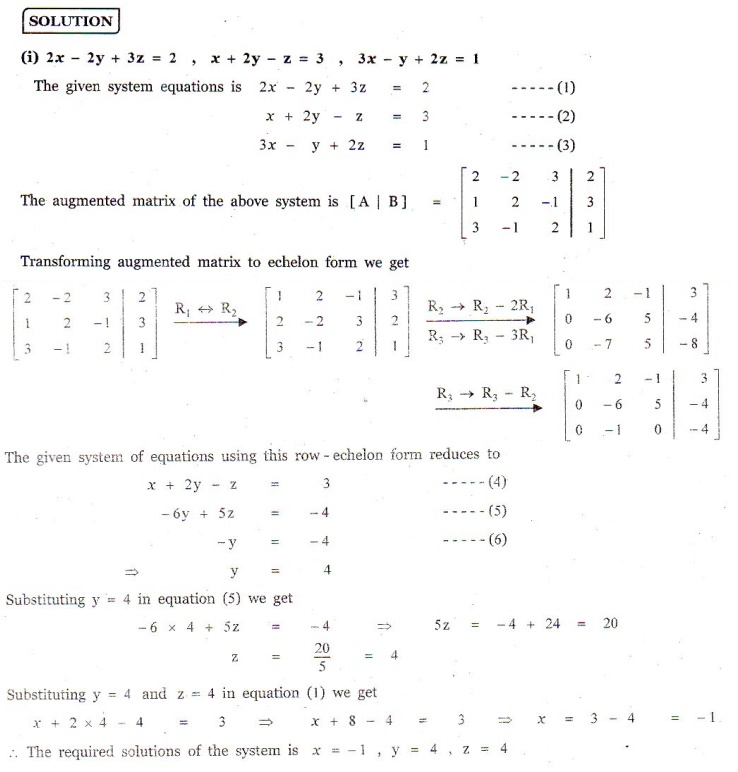Home | | Maths 12th Std | Exercise 1.5: Matrix: Gaussian Elimination Method

# Exercise 1.5: Matrix: Gaussian Elimination Method

Solve the following systems of linear equations by Gaussian elimination method:

EXERCISE 1.5

1. Solve the following systems of linear equations by Gaussian elimination method:

(i) 2x - 2 y + 3z = 2, x + 2 y - z = 3, 3x - y + 2z = 1

(ii) 2x + 4 y + 6z = 22, 3x + 8 y + 5z = 27, - x + y + 2z = 22. If  ax2 + bx + c is  divided  by  x + 3, x - 5 ,  and x -1, the remainders are 21, 61 and 9 respectively. Find a, b and c. (Use Gaussian elimination method.)3. An amount of â‚ą 65,000 is invested in three bonds at the rates of 6%, 8% and 10% per annum respectively. The total annual income is â‚ą 4,800. The income from the third bond is â‚ą 600 more than that from the second bond. Determine the price of each bond. (Use Gaussian elimination method.)4. A boy is walking along the path y = ax2 + bx + c through the points (-6, 8), (-2, -12) , and (3,8) . He wants to meet his friend at P(7, 60) . Will he meet his friend? (Use Gaussian elimination method.)1. (i) x = -1, y = 4, z = 4

(ii) x = 3, y = 1, z = 2

2. a = 2, b = 1, c = 6

3. â‚ą 30000, â‚ą 15000, â‚ą 20000

4. a = 1, b = 3, c = -10 , yes

Tags : Problem Questions with Answer, Solution , 12th Mathematics : UNIT 1 : Applications of Matrices and Determinants
Study Material, Lecturing Notes, Assignment, Reference, Wiki description explanation, brief detail
12th Mathematics : UNIT 1 : Applications of Matrices and Determinants : Exercise 1.5: Matrix: Gaussian Elimination Method | Problem Questions with Answer, Solution